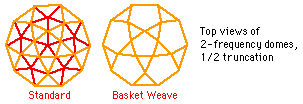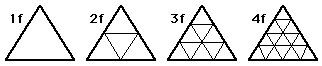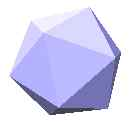A modified geodesic dome design that omits some of the edges, leaving a network of lines that interlock in a pattern of triangles, pentagons, and (in the higher frequencies) hexagons. These domes are made with long, continuous pieces of material (or edges that are joined end-to-end so they act as continuous pieces). The long pieces of material in the dome pattern can be woven over and under each other like strips of wicker in a basket. The basket weave domes use only about half the number of edges of a regular dome, so they are well suited to large, lightweight, portable structures such as tents. The Small PVC Dome and Large PVC Dome are examples of basket weave domes.Dome
A structure consisting of a partial sphere (usually about half of a sphere) used to enclose space. Domes are commonly used for homes, greenhouses, or to enclose equipment such as radar.
Frequency
When talking about domes, frequency refers to the number of pieces that each edge of the base figure is divided into in the process of triangulating its sides. For instance, we might start with a base figure of an icosahedron and divide each edge of each triangular face into 3 equal lengths. Those new points are connected to divide the original triangle into 9 smaller triangles. Since the original edges were divided into 3 parts, we call this a 3-frequency dome. The frequency is commonly abbreviated as "f", so a 2-frequency dome is called 2f, 3-frequency is 3f, and so forth.You can find more about frequency at the Synergetics Home Page section on geodesic dome geometry. In the discussion of Domes come in classes, the figure labeled Class 1shows a triangular face subdivided to 5-frequency. The figure to its right shows an icosahedron triangulated to 3-frequency.
Geodesic
A circular line that goes around the full width of a sphere (like the equator or any of the longitude lines that go all the way around the earth). Geodesic domes get their name because in most of these domes, the edges lie on geodesic lines. Geodesics are also called "great circles" because they are the largest circles you can draw on a sphere.
Golden Proportion
Sometimes called the golden mean or the golden ratio. An irrational number (but then, who's to say what isrational in this crazy world!). It has a special symbol, the Greek letter Tau (or, in some texts, the Greek letter Phi), and is calculated as 1 plus the square root of 5, divided by 2 (approximately equal to 1.618033989). It shows up many places in geometry and nature. For instance, it is the ratio of one of the diagonals of a regular pentagon to the length of its edge.
Icosahedron
(plural: icosahedra) A polyhedron consisting of twenty equilateral triangles which are grouped with 5 triangles around each vertex. Icosahedra are commonly used as a basis for geodesic dome designs because they are fairly round to begin with.Polyhedron
(plural: polyhedra) Any 3-dimensional geometrical figure with many sides. Pyramids, cubes, and geodesic domes are all polyhedra. From the Greek roots poly(meaning many) and hedron(meaning side).
Small Rhombicosidodecahedron
Say thatten times, fast! A 62-sided polyhedron with twelve pentagonal (5-sided) faces, 20 triangular (3-sided) faces, and 30 square (or sometimes rectangular) faces. This is the shape used for the connectors in the Zometool modeling kits.
Triangulation
In a dome context, triangulation is used to mean the process of subdividing a triangle into smaller triangles. So if the base figure (usually an icosahedron) has large triangular faces, then triangulation involves subdividing each of the icosahedron's triangular faces into a grid of smaller triangles. (Note: triangulationis the term I've always used, if anybody knows a more accepted term for this concept, please let me know!) See also Frequency.
Truncation
To "truncate" a polyhedron means to cut off part of it, usually along some natural dividing line (like the middle of a sphere). Here it means cutting a full geodesic sphere off at some "latitude line" to make a dome out of it. For example, cutting the sphere exactly in half at the "equator" results in a dome which is 1/2 of a sphere, which is called a "1/2 truncation."
Vertex
(plural: vertices) The mathematical term for one of the corners of a polyhedron, where the edges come together. At the vertices of a geodesic dome, edges typically come together in groups of 5 or 6.

If there's any terms you don't see here that you think need to be defined (or if the definitions themselves are unclear!), please email Walt with your suggestions. Thanks for your help!